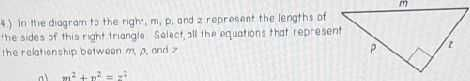### Still have math questions?4.) in the diagranis the righ", $$m , p$$ and $$2$$ repreent the lengths at the sides of this right iriangie select, all the oquations that represent the ralation ship botwaen $$m$$ end $$z$$